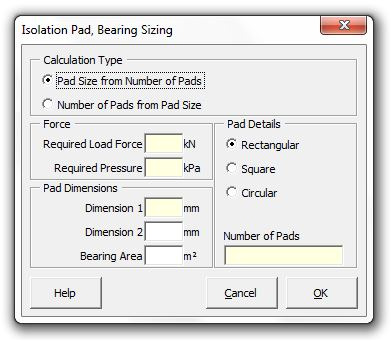### Strutt Help

Isolation Pad, Bearing Sizing   1/1, 1/3

Strutt|Vibration|Isolation Design|Bearing Pad Sizer calculates either the bearing pad size from the known number of pads required or the number of pads required from the known bearing pad size, and inserts the result into the active row in the worksheet.Required Pad Size from Number of Pads calculates the required bearing pad size for a bearing based on achieving a given bearing pressure (P) in a number of bearings (N) based on a given load force (F).
The required bearing area per bearing (A) is calculated using A=(F/P)/N
Once the required bearing area is known, the bearing dimensions are calculated based on the desired bearing shape. For circular or square pads, the pad dimensions may be calculated directly; for rectangular bearings one bearing dimension must be specified.

Number of Pads from Known Pad Size calculates the number of pads (N) of specified size and thus area (A) to achieve a given bearing pressure (P) from an applied force F, using N=F/PA.